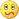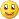# Martingale EA - page 611037

ElectricSavant:
could an input be created to insert a wav file...type it in to the input area? put a default there as an example...but you would need to download the wave file somewhere if it were not microsoft...

Probably, I don't have any experience with that type of coding. Yet...[Deleted]

wolfe you can do anything with code...

wolfe:
Probably, I don't have any experience with that type of coding. Yet...1037

Progress is good

The code is going well. There are a lot of options, and a lot that could go wrong. That's what makes me nervous.Here are all the options right now-

extern double Start_Lot_Size=0.1;//starting lot size for cycle

extern double Lot_Size_Increment=0.1;//Additional orders will increase by this amount

extern bool Double_Lotsize=false;//set to true if you want to just double every lotsize, Lot_Size_Increment ignored if true

extern bool Choose_Own_Progression=false;

extern bool Close_All_Max=false;//select true if you want ALL orders to close if Max_Trades is hit

extern bool Pyramid=true;//if true trades with trend, if false trades against trend

extern bool Auto_Restart=true;//set to true if you want EA to re-start after each cycle

extern bool Use_MA_Entry=false;//select true if you want moving average to decide starting direction

extern int Next_Trade=20;//next trade pip increment if Use_MA_Entry =true (will trade this many pips above OR below last order)

extern int MA_Period=7;//moving average period for calculation

extern int Timeframe=5;//timeframe used for MA calculation, 1=1m, 2=5m, 3=15m, 4=30m, 5=1h, 6=4h, 7=1d

extern bool Use_Kayvan_Method=true;//select to use kayvan's method

extern int Bar_Timeframe=5;//Bar Timeframe used to look back on, 1=1m, 2=5m, 3=15m, 4=30m, 5=1h, 6=4h, 7=1d

extern int Bars_Look_Back=1;//number of bars looked back at from current bar for high and low

extern bool Use_Trailing_Stop=true;//select true to use a trailing stop based on total \$amount

extern double Trail_Start=10;//TS will start after this \$Profit amount is reached

extern double TSLoss_Percent=50;//%Percentage of your HIGHEST profit you can lose before close all is performed

extern bool Close_By_Percent=false;//If true it will close by percent, if false it will close by Profit or Loss in \$\$

extern double TP_Percent=5;//will take profit if profit is greater than percent of account balance (close all orders)

extern double SL_Percent=10;//will stop loss if profit is less than percent of account balance (close all orders)

extern double Close_By_Profit=5;//close if >= to this \$ amount

extern double Close_By_StopLoss=99999;//Amount of money to close all if lost

extern int Slippage=5;//slippage for ordersend command

extern int Number_Of_Tries=5;//Number of tries to send an OrderSend()command if not going through

extern bool Sound_OrderSend=true;//if true a sound will be played when an order goes through

I hope to be done soon. Are there any options I forgot?110

It would be very nice if you also add a "sound - profit taken" or "sound - end of cycle" - just for fun -wolfe:
The code is going well. There are a lot of options, and a lot that could go wrong. That's what makes me nervous.Here are all the options right now-

extern double Start_Lot_Size=0.1;//starting lot size for cycle

extern double Lot_Size_Increment=0.1;//Additional orders will increase by this amount

extern bool Double_Lotsize=false;//set to true if you want to just double every lotsize, Lot_Size_Increment ignored if true

extern bool Choose_Own_Progression=false;

extern bool Close_All_Max=false;//select true if you want ALL orders to close if Max_Trades is hit

extern bool Pyramid=true;//if true trades with trend, if false trades against trend

extern bool Auto_Restart=true;//set to true if you want EA to re-start after each cycle

extern bool Use_MA_Entry=false;//select true if you want moving average to decide starting direction

extern int Next_Trade=20;//next trade pip increment if Use_MA_Entry =true (will trade this many pips above OR below last order)

extern int MA_Period=7;//moving average period for calculation

extern int Timeframe=5;//timeframe used for MA calculation, 1=1m, 2=5m, 3=15m, 4=30m, 5=1h, 6=4h, 7=1d

extern bool Use_Kayvan_Method=true;//select to use kayvan's method

extern int Bar_Timeframe=5;//Bar Timeframe used to look back on, 1=1m, 2=5m, 3=15m, 4=30m, 5=1h, 6=4h, 7=1d

extern int Bars_Look_Back=1;//number of bars looked back at from current bar for high and low

extern bool Use_Trailing_Stop=true;//select true to use a trailing stop based on total \$amount

extern double Trail_Start=10;//TS will start after this \$Profit amount is reached

extern double TSLoss_Percent=50;//%Percentage of your HIGHEST profit you can lose before close all is performed

extern bool Close_By_Percent=false;//If true it will close by percent, if false it will close by Profit or Loss in \$\$

extern double TP_Percent=5;//will take profit if profit is greater than percent of account balance (close all orders)

extern double SL_Percent=10;//will stop loss if profit is less than percent of account balance (close all orders)

extern double Close_By_Profit=5;//close if >= to this \$ amount

extern double Close_By_StopLoss=99999;//Amount of money to close all if lost

extern int Slippage=5;//slippage for ordersend command

extern int Number_Of_Tries=5;//Number of tries to send an OrderSend()command if not going through

extern bool Sound_OrderSend=true;//if true a sound will be played when an order goes through

I hope to be done soon. Are there any options I forgot?[Deleted]

well there was one...only enter when a kayvan entry exceeds its previous entry true or false

LastEntryFilter: default is true. (true means that the next entry must exceed the last entry accoording to if it is a pyramid or an average down choice in the above input. false means that the last entry is ignored and simply enters on the criteria)110

Still.....................

Files:
cable_4.gif  50 kb1037

sivach:
It would be very nice if you also add a "sound - profit taken" or "sound - end of cycle" - just for fun -And which wav sound do we want for this?1037

ElectricSavant:
well there was one...only enter when a kayvan entry exceeds its previous entry true or false LastEntryFilter: default is true. (true means that the next entry must exceed the last entry accoording to if it is a pyramid or an average down choice in the above input. false means that the last entry is ignored and simply enters on the criteria)

Oh yeah! I knew there was something else! I'll do my best to include this option as well.

My brain is getting scrambled!110

Thanks wolf, any wav sound of your choice is good enough.wolfe:
And which wav sound do we want for this?[Deleted]

ok so I can see that doubling or some progression is needed and its not enought to just use 0.1

sivach TY...

do you have any observations you would like to share?

ES

sivach:
Still.....................